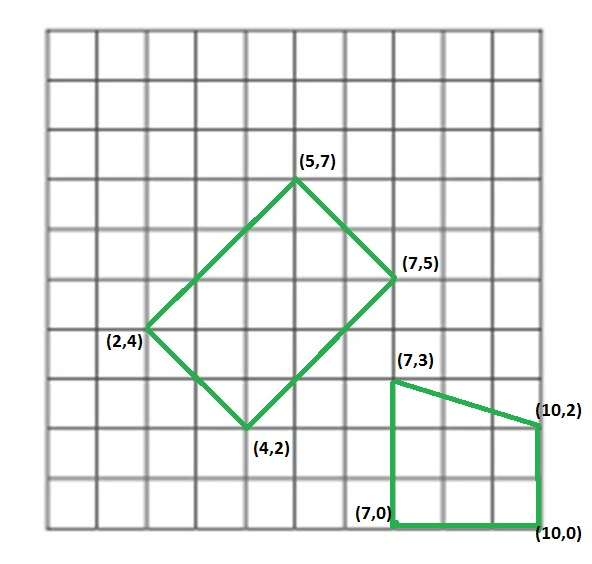# Check if four segments form a rectangle

We are given four segments as a pair of coordinates of their end points. We need to tell whether those four line segments make a rectangle or not.
Examples:

```Input : segments[] =  [(4, 2), (7, 5),
(2, 4), (4, 2),
(2, 4), (5, 7),
(5, 7), (7, 5)]
Output : Yes
Given these segment make a rectangle of length 3X2.

Input : segment[] = [(7, 0), (10, 0),
(7, 0), (7, 3),
(7, 3), (10, 2),
(10, 2), (10, 0)]
Output : Not
These segments do not make a rectangle.

Above examples are shown in below diagram.```

This problem is mainly an extension of How to check if given four points form a square

## Recommended: Please solve it on “PRACTICE ” first, before moving on to the solution.

We can solve this problem by using properties of a rectangle. First, we check total unique end points of segments, if count of these points is not equal to 4 then the line segment can’t make a rectangle. Then we check distances between all pair of points, there should be at most 3 different distances, one for diagonal and two for sides and at the end we will check the relation among these three distances, for line segments to make a rectangle these distance should satisfy Pythagorean relation because sides and diagonal of rectangle makes a right angle triangle. If they satisfy mentioned conditions then we will flag polygon made by line segment as rectangle otherwise not.

 `// C++ program to check whether it is possible  ` `// to make a rectangle from 4 segments  ` `#include   ` `using` `namespace` `std;  ` `#define N 4  ` ` `  `// structure to represent a segment  ` `struct` `Segment  ` `{  ` `    ``int` `ax, ay;  ` `    ``int` `bx, by;  ` `};  ` ` `  `// Utility method to return square of distance  ` `// between two points  ` `int` `getDis(pair<``int``, ``int``> a, pair<``int``, ``int``> b)  ` `{  ` `    ``return` `(a.first - b.first)*(a.first - b.first) +  ` `        ``(a.second - b.second)*(a.second - b.second);  ` `}  ` ` `  `// method returns true if line Segments make  ` `// a rectangle  ` `bool` `isPossibleRectangle(Segment segments[])  ` `{  ` `    ``set< pair<``int``, ``int``> > st;  ` ` `  `    ``// putiing all end points in a set to  ` `    ``// count total unique points  ` `    ``for` `(``int` `i = 0; i < N; i++)  ` `    ``{  ` `        ``st.insert(make_pair(segments[i].ax, segments[i].ay));  ` `        ``st.insert(make_pair(segments[i].bx, segments[i].by));  ` `    ``}  ` ` `  `    ``// If total unique points are not 4, then  ` `    ``// they can't make a rectangle  ` `    ``if` `(st.size() != 4)  ` `        ``return` `false``;  ` ` `  `    ``// dist will store unique 'square of distances'  ` `    ``set<``int``> dist;  ` ` `  `    ``// calculating distance between all pair of  ` `    ``// end points of line segments  ` `    ``for` `(``auto` `it1=st.begin(); it1!=st.end(); it1++)  ` `        ``for` `(``auto` `it2=st.begin(); it2!=st.end(); it2++)  ` `            ``if` `(*it1 != *it2)  ` `                ``dist.insert(getDis(*it1, *it2));  ` ` `  `    ``// if total unique distance are more than 3,  ` `    ``// then line segment can't make a rectangle  ` `    ``if` `(dist.size() > 3)  ` `        ``return` `false``;  ` ` `  `    ``// copying distance into array. Note that set maintains  ` `    ``// sorted order.  ` `    ``int` `distance;  ` `    ``int` `i = 0;  ` `    ``for` `(``auto` `it = dist.begin(); it != dist.end(); it++)  ` `        ``distance[i++] = *it;  ` ` `  `    ``// If line seqments form a square  ` `    ``if` `(dist.size() == 2)  ` `    ``return` `(2*distance == distance);  ` ` `  `    ``// distance of sides should satisfy pythagorean  ` `    ``// theorem  ` `    ``return` `(distance + distance == distance);  ` `}  ` ` `  `// Driver code to test above methods  ` `int` `main()  ` `{  ` `    ``Segment segments[] =  ` `    ``{  ` `        ``{4, 2, 7, 5},  ` `        ``{2, 4, 4, 2},  ` `        ``{2, 4, 5, 7},  ` `        ``{5, 7, 7, 5}  ` `    ``};  ` ` `  `    ``(isPossibleRectangle(segments))?cout << ``"Yes\n"``:cout << ``"No\n"``;  ` `}  `

Output:

```Yes
```

This article is contributed by Utkarsh Trivedi. If you like GeeksforGeeks and would like to contribute, you can also write an article using contribute.geeksforgeeks.org or mail your article to contribute@geeksforgeeks.org. See your article appearing on the GeeksforGeeks main page and help other Geeks.